The Accu-CT® series of current transformers (CTs) meet the accuracy requirements of three commonly used standards:

• ANSI/IEEE C57.13-2008
• IEC 61869-2:2012
• IEC 60044-1 Edition 1.2 (withdrawn)

These CT accuracy standards describe the typical secondary output of a current transformer as 5 amps or 1 amp with an external burden. The Accu-CT ACTL-0750 and ACTL-1250 families have built-in burden resistors and provide voltage outputs (nominally 0.33333 Vac, also available at 1.00 Vac). The transformer correction factor (TCF), accuracy, and phase angle limits of these CT accuracy standards can be applied to the voltage output of the Accu-CT products.

For additional information see Meter Accuracy Standards (AN-136)

## C57.13

The C57.13 standard has different accuracy classes: Class 1.2, Class 0.6, and Class 0.3. Each of these accuracy classes specifies the limit for the TCF as a percentage, so class 1.2 means the CT TCF must be within 1.2% of ideal at 100% of rated primary current.

Because of the way TCF is defined, the resulting gain (accuracy) limits and phase angle limits form a parallelogram when plotted on a graph, which has the effect of allowing larger positive phase angle errors for positive ratio correction factor (RCF) values and larger negative phase angle errors for negative RCF values. The logic behind this is to limit the worst-case system error when using the CT in a metering system with an inductive load having a power factor of 0.6.

For the Accu-CT series, we offer three grades:

### Class 1.2 (Standard)

The standard grade CT meets the IEEE C57.13 accuracy class 1.2 limits as well as tighter accuracy and phase angle limits not required by C57.13.

• TCF: ±1.2% at 100% and 120% of rated primary current
• TCF: ±2.4% at 10% of rated primary current

#### Enhanced Limits Not Required by C57.13

• TCF: ±2.4% at 1% of rated primary current
• Accuracy: ±0.75% from 1% to 120% of rated primary current
• Phase angle: ±0.50 degrees (30 minutes) from 1% to 120% of rated current

### Class 0.6

The higher accuracy grade “Opt C0.6” meets the IEEE C57.13 accuracy class 0.6 limits as well as tighter accuracy and phase angle limits not required by C57.13.

• TCF: ±0.6% at 100% and 120% of rated primary current
• TCF: ±1.2% at 10% of rated primary current

#### Enhanced Limits Not Required by C57.13

• TCF: ±1.2% at 1% of rated primary current
• Accuracy: ±0.50% from 1% to 120% of rated primary current
• Phase angle: ±0.25 degrees (15 minutes) from 1% to 120% of rated current
• (ACTL-0750 models) ±0.50 degrees (15 minutes) below 0°C from 1% to 10% of rated current

### Class 0.3

The higher accuracy grade “Opt C0.3” meets the IEEE C57.13 accuracy class 0.3 limits as well as tighter accuracy and phase angle limits not required by C57.13. Also meets or exceeds IEC 60044-1 and IEC 61869-2 class 0.5S.

• TCF: ±0.3% at 100% and 120% of rated primary current
• TCF: ±1.2% at 10% of rated primary current

#### Enhanced Limits Not Required by C57.13

• TCF: ±1.2% at 1% of rated primary current
• Accuracy: ±0.50% from 1% to 120% of rated primary current
• Phase angle: ±0.25 degrees (15 minutes) from 1% to 120% of rated current
• (ACTL-0750 models) ±0.50 degrees (15 minutes) below 0°C from 1% to 10% of rated current

### Ratio Correction Factor (RCF)

The following definition is provided for informational purposes, but CCS does not typically use the RCF, instead describing the same concept as the current transformer accuracy. CCS does not provide RCF values for our CTs, although the RCF may be computed from the “Measured Accuracy” provided on the Accu-CT Certificate of Calibration.

A correction factor is a number (generally near 1.0) that can be multiplied by the measured value to obtain a corrected value. The ratio correction factor (RCF) is defined as the factor that when multiplied by the current transformer output will yield the correct result: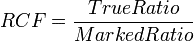$RCF = frac{TrueRatio}{MarkedRatio}$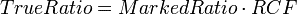$TrueRatio = MarkedRatio cdot RCF$

For example, if a CT is supposed to be a 500:0.33333 Vac CT (500 amp input yields a 0.33333 Vac output), then the “marked ratio” would be 500:0.33333. If the actual output for a 500 amp input was 0.340 Vac (2% high), then the RCF would be:$RCF = frac{500:0.340}{500:0.33333} = frac{0.33333}{0.340} = 0.98038$

Multiplying the full-scale output of 0.340 Vac by 0.98038 yields the corrected full-scale output of 0.33333 Vac.

### Transformer Correction Factor (TCF)

The following definition is provided for informational purposes, but CCS does not typically use the TCF, instead describing CT errors as accuracy and phase angle errors. CCS does provide TCF values on the Accu-CT Certificate of Calibration, but the WattNode® meters do not utilize TCF correction factors.

The transformer correction factor (TCF) is defined for current transformers in the IEEE C57.13 – 2008 standard pp 13-14 as follows.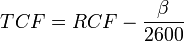$TCF = RCF - frac{beta}{2600}$

• RCF is the Ratio Correction Factor
•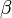$beta$ is the phase angle in minutes (positive for the secondary signal leading the primary current)

Converting this equation to degrees yields: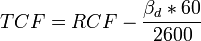$TCF = RCF - frac{beta_d * 60}{2600}$

•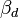$beta_d$ is the phase angle in degrees (positive for the secondary signal leading the primary current)

## 60044-1 and 61869-2

The IEC 60044-1 and IEC 61869-2 accuracy limits are simpler than C57.13, only defining allowed ratio (accuracy) and phase angle errors.

For the Accu-CT, we meet three IEC 60044-1/61869-2 grades. Note: some Accu-CT models are available in 50 Hz versions, optimized for best performance at 50 Hz, so check the datasheet to determine if you should order “Opt 50Hz” for 50 Hz applications.

### Class 1.0 (Standard)

The standard-grade Accu-CT meets or exceeds the accuracy class 1.0 limits.

• Accuracy: ±1.0% at 100% and 120% of rated primary current
• Accuracy: ±1.5% at 20% of rated primary current
• Accuracy: ±3.0% at 5% of rated primary current
• Phase angle: ±1.0 degrees (60 minutes) at 100% and 120% of rated current
• Phase angle: ±1.5 degrees (90 minutes) at 20% of rated current
• Phase angle: ±3.0 degrees (180 minutes) at 5% of rated current

#### Enhanced Limits Not Required by 60044-1/61869-2

The standard-grade CT also meets our more stringent limits, which exceed the class 1.0 requirements.

• Accuracy: ±0.75% from 1% to 120% of rated primary current
• Phase angle: ±0.50 degrees (30 minutes) from 1% to 120% of rated current

### Class 0.5 and Class 0.5S

The higher accuracy grade “Opt C0.6” and “Opt C0.3” CTs meet the class 0.5 and 0.5S (extended range) limits.

• Accuracy: ±0.50% at 20, 100%, and 120% of rated primary current
• Accuracy: ±0.75% at 5% of rated primary current
• Accuracy: ±1.50% at 1% of rated primary current
• Phase angle: ±0.50 degrees (30 minutes) at 20%, 100%, and 120% of rated current
• Phase angle: ±0.75 degrees (45 minutes) at 5% of rated current
• Phase angle: ±1.50 degrees (90 minutes) at 1% of rated current

#### Enhanced Limits Not Required by 60044-1/61869-2

The “Opt C0.6” and “Opt C0.3” grade CTs meet our more stringent limits, which exceed the class 0.5 and 0.5S requirements.

• Accuracy: ±0.50% from 1% to 120% of rated primary current
• Phase angle: ±0.25 degrees (15 minutes) from 1% to 120% of rated current;
(ACTL-0750 models) ±0.50 degrees (15 minutes) below 0°C from 1% to 10% of rated current

### Class 0.2 and Class 0.2S

The higher accuracy grade “ACTL-1250 Opt C0.2” CTs meet the class 0.2 and 0.2S (extended range) limits.

• Accuracy: ±0.20% at 20, 100%, and 120% of rated primary current
• Accuracy: ±0.35% at 5% of rated primary current
• Accuracy: ±0.75% at 1% of rated primary current
• Phase angle: ±0.167 degrees (10 minutes) at 20%, 100%, and 120% of rated current
• Phase angle: ±0.25 degrees (15 minutes) at 5% of rated current
• Phase angle: ±0.50 degrees (30 minutes) at 1% of rated current

#### Enhanced Limits Not Required by 60044-1/61869-2

The “Opt C0.2” grade CTs meet our more stringent limits, which exceed the class 0.2 and 0.2S requirements.

• Accuracy: ±0.20% from 10% to 120% of rated primary current
• Accuracy: ±0.30% from 1% to 9% of rated primary current
• Phase angle: ±0.125 degrees (7.5 minutes) from 10% to 120% of rated current
• Phase angle: ±0.250 degrees (15 minutes) from 1% to 9% of rated current